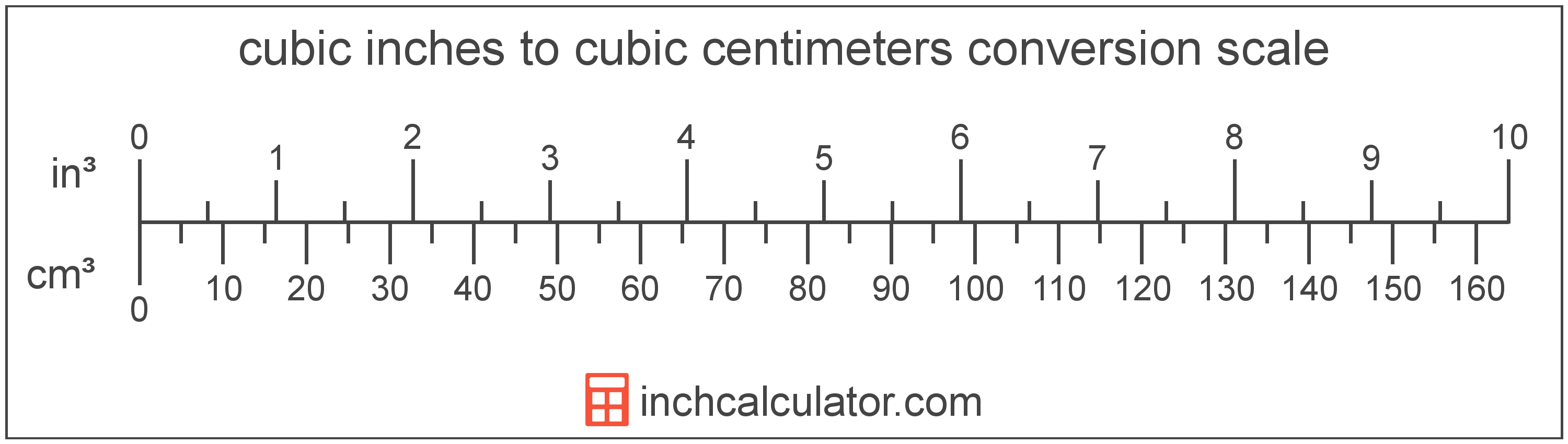# Cubic Inches to Cubic Centimeters Conversion

Enter the volume in cubic inches below to get the value converted to cubic centimeters.

Results in Cubic Centimeters:1 in³ = 16.387064 cm³

## How to Convert Cubic Inches to Cubic CentimetersTo convert a cubic inch measurement to a cubic centimeter measurement, multiply the volume by the conversion ratio.

Since one cubic inch is equal to 16.387064 cubic centimeters, you can use this simple formula to convert:

cubic centimeters = cubic inches × 16.387064

The volume in cubic centimeters is equal to the cubic inches multiplied by 16.387064.

For example, here's how to convert 5 cubic inches to cubic centimeters using the formula above.
5 in³ = (5 × 16.387064) = 81.93532 cm³

## Cubic Inches

A cubic inch is a unit of volume equal to the space consumed by a cube with sides that are one inch in all directions. One cubic inch is equivalent to about 16.387 cubic centimeters or 0.554 fluid ounces.

The cubic inch is a US customary and imperial unit of volume. A cubic inch is sometimes also referred to as a cubic in. Cubic inches can be abbreviated as in³, and are also sometimes abbreviated as cu inch, cu in, or CI. For example, 1 cubic inch can be written as 1 in³, 1 cu inch, 1 cu in, or 1 CI.

## Cubic Centimeters

The cubic centimeter is a unit that is equal to the volume of a cube with one centimeter sides. One cubic centimeter is equal to about 0.061 cubic inches.

The cubic centimeter, or cubic centimetre, is a multiple of the cubic meter, which is the SI derived unit for volume. In the metric system, "centi" is the prefix for 10-2. Cubic centimeters can be abbreviated as cm³, and are also sometimes abbreviated as cu cm, cc, or ccm. For example, 1 cubic centimeter can be written as 1 cm³, 1 cu cm, 1 cc, or 1 ccm.

## Cubic Inch to Cubic Centimeter Conversion Table

Cubic inch measurements converted to cubic centimeters
Cubic Inches Cubic Centimeters
1 in³ 16.39 cm³
2 in³ 32.77 cm³
3 in³ 49.16 cm³
4 in³ 65.55 cm³
5 in³ 81.94 cm³
6 in³ 98.32 cm³
7 in³ 114.71 cm³
8 in³ 131.1 cm³
9 in³ 147.48 cm³
10 in³ 163.87 cm³
11 in³ 180.26 cm³
12 in³ 196.64 cm³
13 in³ 213.03 cm³
14 in³ 229.42 cm³
15 in³ 245.81 cm³
16 in³ 262.19 cm³
17 in³ 278.58 cm³
18 in³ 294.97 cm³
19 in³ 311.35 cm³
20 in³ 327.74 cm³
21 in³ 344.13 cm³
22 in³ 360.52 cm³
23 in³ 376.9 cm³
24 in³ 393.29 cm³
25 in³ 409.68 cm³
26 in³ 426.06 cm³
27 in³ 442.45 cm³
28 in³ 458.84 cm³
29 in³ 475.22 cm³
30 in³ 491.61 cm³
31 in³ 508 cm³
32 in³ 524.39 cm³
33 in³ 540.77 cm³
34 in³ 557.16 cm³
35 in³ 573.55 cm³
36 in³ 589.93 cm³
37 in³ 606.32 cm³
38 in³ 622.71 cm³
39 in³ 639.1 cm³
40 in³ 655.48 cm³

## References

1. National Institute of Standards and Technology, Specifications, Tolerances, and Other Technical Requirements for Weighing and Measuring Devices, Handbook 44 - 2019 Edition, https://nvlpubs.nist.gov/nistpubs/hb/2019/NIST.HB.44-2019.pdf
2. National Institute of Standards and Technology, Specifications, Tolerances, and Other Technical Requirements for Weighing and Measuring Devices, Handbook 44 - 2019 Edition, https://nvlpubs.nist.gov/nistpubs/hb/2019/NIST.HB.44-2019.pdf